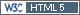## 'whatif' plotting from 4X2 to 2X2 [Power / Sample Size]

Dear Helmut, Dear Zizou,

thank you for pointing out this interesting case!
I tried to write some code to see what could we expect with given variabilities from full replicate dataset.
May be it is not so fair since I'm sampling from given data, not from CV directly.
By the way some clues to the question 'what if'

```library(dplyr) library(ggplot2) alpha <- 0.05 nsims <- 1000 Sample2from4 <- function(Data, datacol = "data", nsims = 1000, alpha = 0.05) {   # columns should be: subject, sequence, period, treatment; data column could be changed in function args   ow    <- options()   options(contrasts = c("contr.treatment", "contr.poly"), digits = 12)   Data <- as.data.frame(Data)   Data\$subject <- as.factor(Data\$subject)   Data\$sequence <- as.factor(Data\$sequence)   Data\$period <- as.numeric(Data\$period)   Data\$treatment <- as.factor(Data\$treatment)   Data\$data <- as.numeric(Data[, match(datacol, colnames(Data))])   SamplingResults <-     data.frame(       PE = numeric(0),       lowerCI = numeric(0),       upperCI = numeric(0),       CV = numeric(0))   for (i in 1:nsims) {     # sampling N          1   2  3   4     # periods to include 12 34  14  23     Data2periods <-       Data %>%       group_by(subject) %>%       mutate(periodsample = sample(1:4, 1)) %>%       filter((periodsample == 1 & (period == 1 | period == 2)) |                (periodsample == 2 & (period == 3 | period == 4)) |                (periodsample == 3 & (period == 1 | period == 4)) |                (periodsample == 4 & (period == 2 | period == 3))       ) %>%       mutate(periodrank = rank(period))     Data2periods\$period <- as.factor(Data2periods\$periodrank)     mod <- lm(log(data) ~ sequence + treatment + period + subject %in% sequence,          data = Data2periods)     PE <- as.numeric(exp(coef(mod)["treatmentT"]))     CI <- exp(confint(mod, "treatmentT", level = 1 - 2 * alpha))     mse <- summary(mod)\$sigma ^ 2     CV <- sqrt(exp(mse) - 1)     SamplingResults <-       rbind(SamplingResults,             data.frame(               PE = PE,               lowerCI = CI,               upperCI = CI,               CV = CV))   }   options(ow) # restore options   return(SamplingResults) } plotSample2from4 <- function(SamplingResults, Data, datacol = "data"){   ow    <- options()   options(contrasts = c("contr.treatment", "contr.poly"), digits = 12)   Data <- as.data.frame(Data)   Data\$subject <- as.factor(Data\$subject)   Data\$sequence <- as.factor(Data\$sequence)   Data\$period <- as.factor(Data\$period)   Data\$treatment <- as.factor(Data\$treatment)   Data\$data <- as.numeric(Data[, match(datacol, colnames(Data))])   Data.modR <- lm(log(data) ~ sequence + subject%in%sequence + period,                   data=Data[Data\$treatment=="R",])   Data.CVwR  <- sqrt(exp(summary(Data.modR)\$sigma ^ 2) - 1)   Data.modT <- lm(log(data) ~ sequence + subject%in%sequence + period,                   data=Data[Data\$treatment=="T",])   Data.CVwT  <- sqrt(exp(summary(Data.modT)\$sigma ^ 2) - 1)   Data.CV <- cbind.data.frame(CV = c(Data.CVwR, Data.CVwT), CVw = c("CVwR", "CVwT") )   MoreThanCVwR <- length(SamplingResults\$CV[SamplingResults\$CV<Data.CVwR]) / length(SamplingResults\$CV)   MoreThanCVwT <- length(SamplingResults\$CV[SamplingResults\$CV<Data.CVwT]) / length(SamplingResults\$CV)   Data.CV <- cbind.data.frame(Data.CV, MoreThanCVw = c(MoreThanCVwR, MoreThanCVwT))   ribbon <- ggplot_build(ggplot() + geom_density(data = SamplingResults, aes(x = CV), colour = "<CVw>"))\$data[]   ribbon\$colour[ribbon\$x<Data.CVwR & ribbon\$x < Data.CVwT ] <- "<CVwR & <CVwT"   ribbon\$colour[ribbon\$x>Data.CVwR & ribbon\$x > Data.CVwT ] <- ">CVwR & >CVwT"   title <- paste0("CVwR = ", sprintf("%.2f%%", Data.CVwR*100),                   ", CVwT = ", sprintf("%.2f%%", Data.CVwT*100),                   "; \n2X2 Study CV > CVwR in ", sprintf("%.2f%%", 100-MoreThanCVwR*100),                   "; 2X2 Study CV > CVwT in ", sprintf("%.2f%%", 100-MoreThanCVwT*100))   densityplot <-     ggplot() +     geom_ribbon(data = ribbon, aes(x = x, ymin = 0, ymax = y, fill = colour), alpha = .4) +     geom_vline(data = Data.CV,  aes(xintercept = CV, color = CVw, linetype = CVw)) +     theme_bw() +     ggtitle(title)+     labs(x="CV", y = "density")   print(densityplot)   return(Data.CV)   options(ow) # restore options }```

now using the dataset from Helmut's post above:
```SamplingResults <- Sample2from4(Data = Data, datacol = "data", nsims = 1000) plotSample2from4(Data = Data, SamplingResults = SamplingResults, datacol = "data")```Amazing! CVwR and CVwT are far away from mean/median!

What about other examples? EMA full replicate:
```SamplingResults <- Sample2from4(Data = ds01, datacol = "PK", nsims = 1000) plotSample2from4(Data = ds01, SamplingResults = SamplingResults, datacol = "PK")```Looks reasonable. Somewhere between CVwR and CVwT. By the way I would not go with pooled CV for 2X2 knowing this...

Kind regards,
Mittyri

Bioequivalence and Bioavailability Forum |  Admin contact
19,302 posts in 4,102 threads, 1,316 registered users;
online 10 (1 registered, 9 guests [including 8 identified bots]).

Restlessness is discontent –
and discontent is the first necessity of progress.
Show me a thoroughly satisfied man –
and I will show you a failure.    Thomas Alva Edison

The BIOEQUIVALENCE / BIOAVAILABILITY FORUM is hosted byIng. Helmut Schütz